### 九年级数学上册第4章《一元二次方程》4.3 用公式法解一元二次方程（第二课时）

• 同步课程
• 本节重点
• 主讲老师

4.3 用公式法解一元二次方程

x²+3=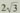x.

x²-x+3=0，
a=1，b=-，c=3.

∵b²-4ac=（-）²-4×1×3=0，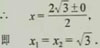（x+1）（3x-1）=1.

3x²+2x-2=0，

∵b²-4ac=2²-4×3×（-2）=28＞0，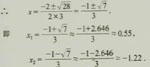1.用公式法解下列方程：
（1）x²-12x+20=0；（2）2x²+11x+5=0；
（3）5x²-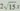+3=0；  （4）5x²+10x-6=0.
2.用公式法解方程2x²-6x+3=0，并求根的近似值（精确到0.01）。
3.一个三角形的两边长分别是8和6，第三边的长是一元二次方程x²-16x+60=0的一个实数根，求该三角形的面积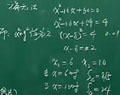4.已知实数x，y满足（x²+y²）（x²+y²-1）=2，试求x²+y²的值。
（x²+y²）（x²+y²-1）=2

a·（a-1）=2
a²-a=2
a²-a+1/4=2+1/4
（a-1/2）²=9/4
a-1/2=±3/2
∴a1=2 a2=-1